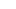# JEST 2020 PhD entrance exam: Physics Syllabus and Exam PatternJEST 2020 PhD entrance exam

The Notification has been passed for Joint Entrance Screening Test (JEST) an entrance for admission to a Ph.D. or Integrated Ph.D. program in physics or theoretical in various other subjects which shall be carried on February 16, 2020. The one-year score of the JEST exam shall be taken into consideration.

Important Dates:

 Particulars Dates The closing date for submitting online applications and payments is extended to 21 Dec 2019 JEST 2020 will be carried on 16 February 2020 Online registrations November 11, 2019, to December 21, 2019 Declaration of JEST result March, 2020

Exam pattern:

These are the main area of exams-

• Analytical Reasoning and its Deduction,
• Combinatorics,
• Data Structures and its Algorithms,
• Discrete math’s,
• Graph and its theory
• Principles of various Programming.

The official website to get more details on the JEST 2020 examination is https://www.jest.org.in/joint-entrance-screening-test .

The physics syllabus is as follows-

Mathematical Methods:

The chapters include-

• Vector Algebra and Vector Calculus,
• Tensors,
• Curvilinear Coordinate Systems,
• Linear Algebra;
• Linear Differential Equations,
• Elements of Sturm-Liouville Theory;
• Special Functions;

Classical Mechanics:

The chapters include-

• Newton’s Rules,
• Conservation of Energy and Momentum,
• Collisions;
• Generalized Coordinates,
• The Principle of Least Action,
• Lagrangian and Hamiltonian Formulations of Mechanics;
• Symmetry and Conservation Laws;
• Nuclear Force Problem,
• Kepler Problem;
• Small Oscillations and Normal Modes;

Electromagnetism & Optics:

The chapters include-

• Electrostatics and Magnetostatics,
• Boundary value and related Problems,
• Multipole and Expansion;
• Fields in Conducting,
• Diamagnetic and Paramagnetic Media;
• Displacement Current;
• Maxwell’s and Equations;
• Energy and Momentum and its Electromagnetic Fields;
• Propagation of Plane and Electromagnetic waves,

Quantum Mechanics:

The chapters include-

• Uncertainty Principle;
• Schrodinger Equation;
• Cepotentials,
• Hydrogen Atom;
• Orbital and Spin Angular Momenta,
• The Addition of Angular Momenta;
• Matrix Formulation of Quantum Theory,
• Unitary Transformations,

Electronics:

The chapters include-

• Basics of semiconductor;
• P-n junctions,
• Diodes,
• Transistors;
• TCR Circuits,
• Amplifiers

Thermodynamics and Statistical Physics:

The chapters include-

• Laws of Thermodynamics,
• Work and Heat,
• Thermodynamic Potentials;
• Elements of Kinetic Theory;
• Maxwell’s Relations;
• Statistical Ensembles;
• Classical Ideal Gas,